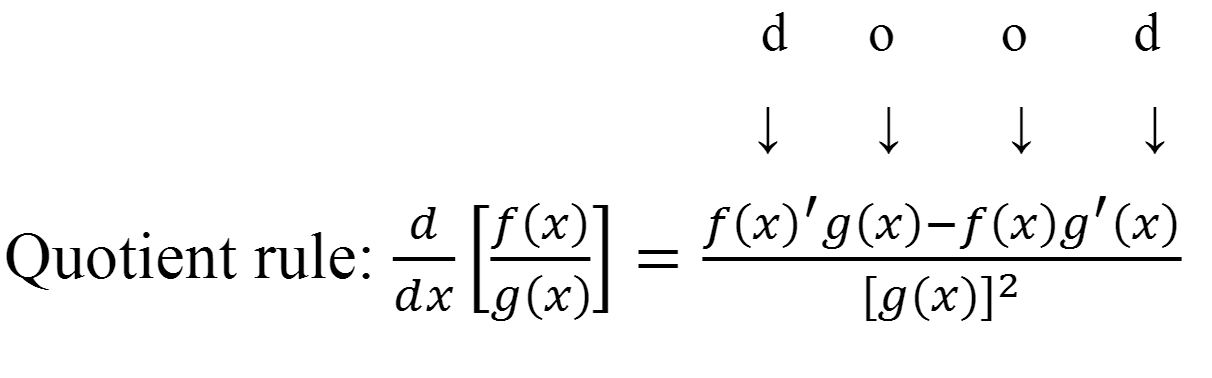# Quotient rule - Derivatives

### Quotient rule

To find the derivative of a function resulted from the quotient of two distinct functions, we need to use the Quotient Rule. In this section, we will learn how to apply the Quotient Rule, with additional applications of the Chain Rule. We will also recognize that the memory trick for the Quotient Rule is a simple variation of the one we used for the Product Rule ("d.o.o.d").

#### Lessons

######